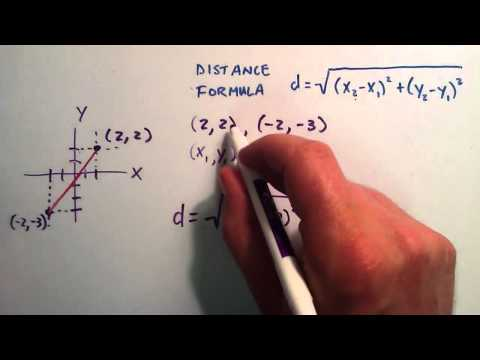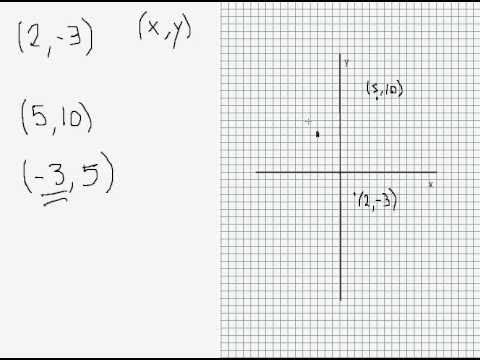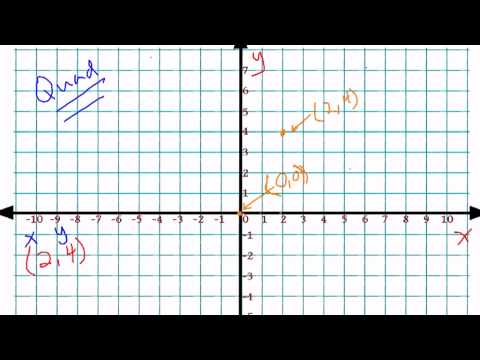## Co-ordinate Geometry

Subject: Optional Maths

#### Overview

Coordinate geometry is a branch of mathematics which deals with the combination of algebra and geometry.This note gives information about the coordinate geometry and describing the cartesian coordinate plane.
##### Co-ordinate Geometry

Introduction:

Rene Descartes is a famous mathematician who has invented the concept of coordinate geometry in 17thcentury . Algebra and Geometry is an overlapping portion which we now say Co-ordinate Geometry . Co-ordinate Geometry is a branch of mathematics which deals with the combination of algebra and geometry .Note;it deals with the exact location of a point ,line and surface.

Things to know; we need to know how we locate a point on a number line which will be comfortable with a plane . the line below is a number line .Here we are standing at 0 and right side of 0 is a positive and left side of 0 is negative .

Describing the cartesian coordinate plane .

Here, a cartesian coordinate plane is also known as a rectangular coordinate system . Which is made up of two number lines and it forms axes .1. The horizontal number line is the X-axis .It is also called abscissa .
2. The vertical number lines are the Y- axis . It is also known as ordinate .
3. The two line intersecting part is called Origin . At this point, both number lines are zero .

Axes divided into four quadrants which marked on the graph with roman numerals.

Each point on the graph is associated with an ordered pair . On the plain figure, the point can be described by its X and Y coordinates .Here coordinates are written as the pair : ( X , Y ). When dealing with an ( x , y ), x and y coordinates are always first and second respectively . There are two values in the ordered pair, so we should be careful that it associates to only one point on the graph .In the graph , we have point A and to know the point A let's look at the origin . So, to reach Awe move four (4) steps to the right and stop . Again we need to move two ( 2 ) step up to get the exact point A . Hence, the coordinate of point A in ( 4 , 2 )As its took us 4 steps on the right and 2 steps above to reach the point .Here x-axis has elongated both ways .So, we can represent it by XX'; where X represents positive and X' represents negative side . Same ways Y axis is elongated up and down both forming YY' where Y represents positive and Y' representing negative side .

As we know, from theory and examples that four quadrants with the help of two axis quadrant I, quadrant II, quadrant III, quadrant IV, respectively .Even can generalises the nature of X' s and Y' s and studies their nature as below.

 Quadrant X-coordinate y-coordinate Nature I positive positive ( a, b ) II positive positive (-a,b ) III positive positive (-a,-b) IV positive positive (a,- b )

Similarly,

All the points on the x-axis will be of nature ( a, 0 ) if it is on the right side of the origin and (-a, 0 ) if it is on the left side of the origin . On the other hand, all the points on the y-axis will be of nature ( 0, b ) if it is above origin and ( 0, -b ) if is below the origin .

Distance Between Two Points:

The distance between two points can be determined by how much we have moved it. If the points are being at the axes it becomes easy for us but if we are moving arbitrarily we need to think. The following example illustrates it: (Figure)

##### Things to remember
• A rectangular coordinate is made up of two number lines.
• The horizontal number line is x- axis  and vertical number line is y- axis.
• x-axis  and y-axis  also known as abcissa and ordinate respectively.
• It includes every relationship which established among the people.
• There can be more than one community in a society. Community smaller than society.
• It is a network of social relationships which cannot see or touched.
• common interests and common objectives are not necessary for society.
##### Videos for Co-ordinate Geometry##### How to Find the Distance Between Two Points - How to Use the Distance Formula##### Introducing the Cartesian Coordinate Plane##### Lesson 2-6: Graphing in Four Quadrants (plotting ordered pairs)

Rene Descrates is  the founder of coordinate geometry .

The vertical line is known as x-axis .

The horizontal line is known as the y-axis.

The point where x-axis and y-axis meet is called origin .

Co-ordinate Geometry is a branch of mathematics which deals with the combination of algebra and geometry .

Solution

X(-3, 2), Y(-1, 2) and Y(4,2) represents a triangle. When measured with a scale two sides XY and YZ are equal so it presents an isosceles triangle.

Solution

According to the question,

O(0,0), Q(4,0) and R(0,5)  is  representing a triangle as it has 3 sides and it is a  right angled triangle as ∠ROQ is 90º as measured with protector.

Solution:

A(1,-1), B(7,3), C(4,6) & D(-2, 2) represents a quadrilateral as it has four sides. Since, the opposite sides are equal it represents a rectangle.

Solution:

Since point P corresponds to 2 on the x-axis and -3 on the y-axis, then P 's ordered pair is coordinate is (2, -3).

Solution:

P(0,0) and Q(6, 0)

Here, let P(0, 0) be (x1, y1) and Q(6, 0) be (x2, y2)

Now, x- component = x2 - x1

= 6 - 0

= 6

y- component = y2 - y1

= 0 - 0

= 0

Here, PQ = 6 units (As it has travelled is only one axis )

Solution:

P(-5,0) and Q(2, 0)

Here, let P(-5, 0) be (x1, y1) and Q(2, 0) be (x2, y2)

Now, x- component = x2 - x1

= 2 -(- 5)

= 2 + 5

= 7

y- component = y2 - y1

= 0 - 0

= 0

Here, PQ = 7 units (As it has travelled is only one axis )

Solution:

P(0,5) and Q(0, -2)

Here, let P(0, 5) be (x1, y1) and Q(0, -2) be (x2, y2)

Now, x- component = x2 - x1

= 0- 0

= 0

y- component = y2 - y1

= -2 - 5

= -7

Here,  Since PQ  only moves along y-axis  PQ= 7 units (distance is never negative)

Solution:

P(1,2) and Q(5, 4)

Here, let P(1, 2) be (x1, y1) and Q(5, 4) be (x2, y2)

Now, x- component = x2 - x1

= 5 - 1

= 4

y- component = y2 - y1

= 4- 2

= 2

Now, distance between PQ is given by,

(PQ)2 = (x component)2 + (y component)2

=  (4)2 + (2)2

= 16 +4

= 20

(PQ) = $\sqrt{20}$

∴ PQ = $\sqrt{20}$ units

Solution:

Here, for OA,

O(0,0) be (x1, y1)

A(2, 2) be (x2, y2)

Now x- component= x1 - x2

= 2 - 0

= 2

y- component = y2 - y1

= 2- 0

= 2

( OA)2  = (x- component)2 + (y- component)2

= (2)2 + (2)2

= 4 + 4

= 8

OA = $\sqrt{8}$ units.

Again, for OB,

O(0,0)  be (x1, y1)

B(-2, -2) be (x2, y2)

Now,

( OB)2  = (x- component)2 + (y- component)2

= (-2)2 + (-2)2

= 4 + 4

= 8

OA = $\sqrt{8}$ units.

Hence, OA= OB  Proved.

Solution

P(1,2) and Q(3, 2)

Here, let P(1, 2) be (x1, y1) and Q(3, 2) be (x2, y2)

Now, x- component = x2 - x1

= 3 - 1

= 1

y- component = y2 - y1

= 2 - 2

= 0

Here, PQ = 1 units (As it has travelled is only one axis )